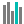#STANDARDIZE (PQL - xl)

This function returns a normalized value from a distribution characterized by mean and standard deviation

• Library: PQL \ Spreadsheet \ Statistical
• Compatibility: Any content (regardless of data source) in the Tabulate spreadsheet module

#### Syntax

STANDARDIZE(x, mean, standard dev)

##### Function Arguments
 Name Description Type Optional x Value to normalize Number mean Arithmetic mean of the distribution Number standard dev Standard deviation of the distribution Must be greater than zero Number